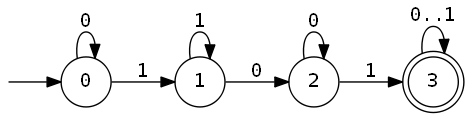# Acknowledgements

This article is a rewrite of the work of Mark Engelberg in his automata repository - with a tweak:

All the code snippets of this page are live and interactive powered by the klipse plugin:

1. Live: The code is executed in your browser
2. Interactive: You can modify the code and it is evaluated as you type

In his repo, Mark shows how to use regular expressions and automata to solve programming riddles. In this article, we will focus on automata.# A hard problem - maximum non-segment sum

In Regular expressions to solve programming interview riddles, we introduced this (hard) problem:

Given a sequence of numbers, find the maximum sum among subsequences that are not a continguous segment.

For example, the result for `[-1 4 5 -3 -4]` should be `5` because `4+5+-4 = 5`, and those three numbers don’t form a segment.

We can’t choose just `4`, or just `5`, or `4+5`, because singletons and adjacent pairs are considered a segment. We can’t even choose the “empty” subsequence with a value of `0`, because that is also considered a segment. We could have chosen things like `-1+5` or `5+-4` or `4+-3`, but they happen to be not as good.

We showed in the article how to solve this problem using brute force and Regular expressions.

# Equivalence between Regular expressions and Finite State Machines

Now, we are going to show an effective solution to this problem, using automata (aka Finite State Machines or FSM).

The basic theorem of automata theory is Kleene’s theorem that states:

Regular expressions are equivalent to Finite State machines

Inspired by this theorem, we can convert our regular expression into a finite state machine.

The regular expression to identify non-segments was:

`/0*1+0+1(0|1)/`

What this regex says is that a non segment bitmask is a sequence of:

• 0 or `0`s
• 1 or more `1`s
• 1 or more `0`s
• a single `1`
• `0`s or `1`s freely

With a bit of thinking, here is the equivalent state machine:# Efficient Algorithm

We can get a `O(n)` algorithm by cleverly interleaving the automaton constraint checking with the summing/max process. As we do one pass through the sequence, our accumulator tracks the maximum sum we can get ending in a given state of our state machine. At the end of the pass, the value associated with the accepting state is our answer.

First, let’s describe our state machine transitions as a clojure map:

``````(def transitions
{:q0 {0 :q0, 1 :q1},
:q1 {0 :q2, 1 :q1},
:q2 {0 :q2, 1 :q3},
:q3 {0 :q3, 1 :q3}})
``````

Clojure maps behave as functions, so it’s very handy move through the state machine.

For instance, when we are at state `:q0` and then input is `1`, we move to state `:q1`:

``````((transitions :q0) 1)
``````

We are going to explore all the states and track all the maximal values of the corresponsing subsequence maximum, using the following rules:

• when the input is `0`, the sum is not modified
• when the input is `1`, we add the corresponding values of the sequence to the sum
``````(defn update [[state sum] x]
(let [state-0 ((transitions state) 0)
state-1 ((transitions state) 1)]
(merge-with max {state-0 sum} {state-1 (+ sum x)})))

``````

The trick is that we don’t need to track all the possible values, we can keep only the maximal ones. In other words, we can merge the resulting `map` into the previous one using `max`.

Let’s try to write `maximum-non-segment-sum` using `map` and `reduce`:

``````(defn maximum-non-segment-sum [s]
(let [max-by-states (reduce (fn [res x]
(apply merge-with max res (map #(update % x) res)))
{:q0 0}  s)]
(:q3 max-by-states)))
``````

Let’s see it in action:

``````(maximum-non-segment-sum [1 2 3 -4 5 -8 4])
``````

You can increase the length of the sequence with no fear: the complexity of this algorithm is `O(n)`!

``````(def n 1000)
(maximum-non-segment-sum (repeatedly n #(- (rand-int 11) 5)))
``````

If you like this article, you will enjoy a lot Mark Engelberg’s talk on youtube about automata.